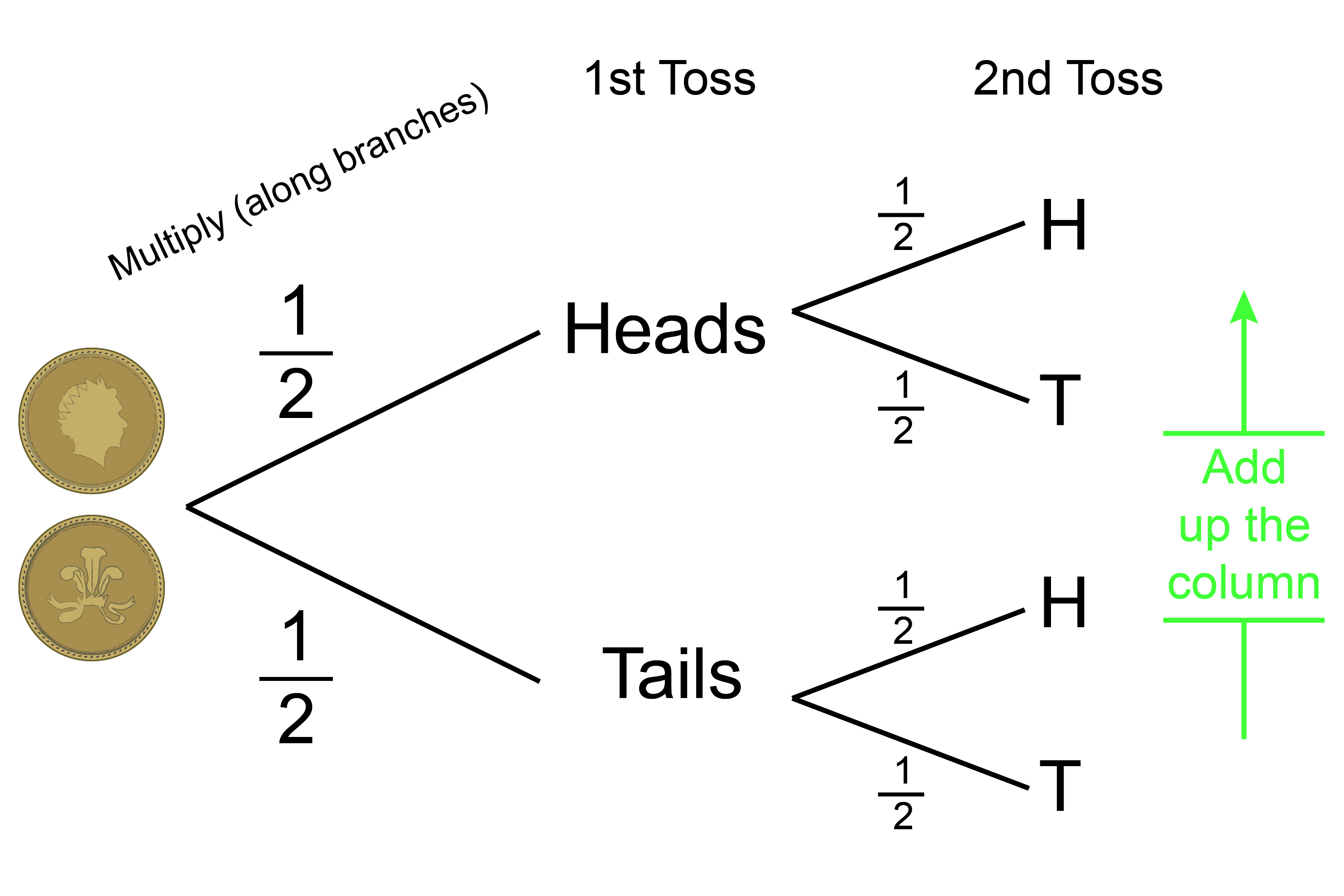# Probability summary

1. Probability\=(Right)/(All) =(The\  \n\umber\ of\ ways\ of \ ac\hiev\i\ng\ suc\c\ess)/(T\he\ \t\otal\ n\umber\ of \ possibl\e\ outcomes

2.  With a probability question:

ALWAYS draw a probability TREE

3.  Probability tree think flicking a coin twice:## Vocabulary you need

1.  Dependent – one event INFLUENCES the outcome of the other (sometimes called conditional probability). Dependent probability is about INFLUENCE.

2.  Independent – one event is NOT influenced by the other. Independent probability is about NOT having an INFLUENCE.

3.  Mutually exclusive – events that can’t both happen at the same TIME.

4.  Non-mutually exclusive – events that can both happen at the same TIME.